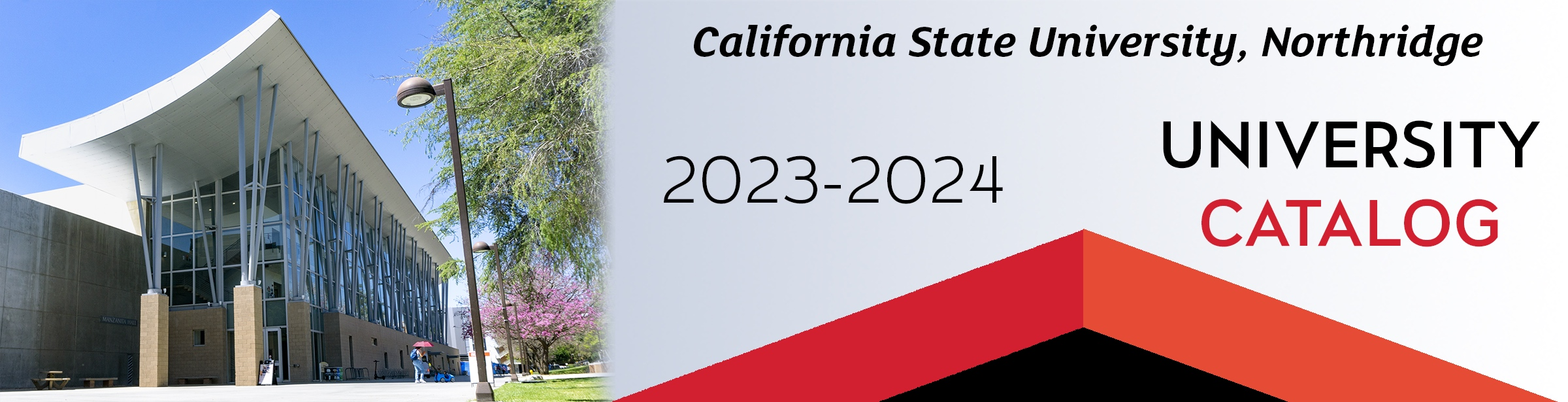## Course: MATH 106. Mathematical Foundations for Non-Calculus Physics (5)

Prerequisites: Listed in Table 1. Mathematics applicable to problems in non-calculus based physics. Sets, inequalities; functions and graphs: polynomial, rational, exponential, logarithmic, trigonometric; introduction to vectors, angular velocity, and parametric equations. This course is not open to students who have credit in MATH 105 or MATH 255A. (Available for General Education, Basic Skills B4 Mathematics and Quantitative Reasoning.)

### MATH 106

Class NumberLocationDayTime